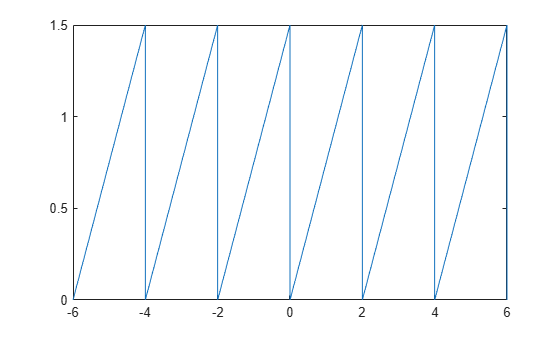mod

Symbolic modulus after division

Starting in R2020b, mod no longer finds the modulus for each coefficient of a symbolic polynomial. For more information, see Compatibility Considerations.

Description

example

m = mod(a,b) finds the modulus after division. To find the remainder, use rem.

If a is a polynomial expression, then mod(a,b) returns the unevaluated modulus of the polynomial.

Examples

collapse all

Find the modulus after division when both the dividend and divisor are integers.

Find the modulus after division for these numbers.

m = [mod(sym(27),4), mod(sym(27),-4), mod(sym(-27),4), mod(sym(-27),-4)]
m = $\left(\begin{array}{cccc}3& -1& 1& -3\end{array}\right)$

Find the modulus after division when the dividend is a rational number, and the divisor is an integer.

Find the modulus after division for these numbers.

m = [mod(sym(22/3),5), mod(sym(1/2),7), mod(sym(27/6),-11)]
m =

$\left(\begin{array}{ccc}\frac{7}{3}& \frac{1}{2}& -\frac{13}{2}\end{array}\right)$

Find the modulus after division when the dividend is a polynomial expression, and the divisor is an integer. If the dividend is a polynomial expression, then mod returns a symbolic expression without evaluating the modulus.

Find the modulus after division by $10$ for the polynomial ${x}^{3}-2x+999$.

syms x
a = x^3 - 2*x + 999;
mUneval = mod(a,10)
mUneval =

To evaluate the modulus for each polynomial coefficient, first extract the coefficients of each term using coeffs.

[c,t] = coeffs(a)
c = $\left(\begin{array}{ccc}1& -2& 999\end{array}\right)$
t = $\left(\begin{array}{ccc}{x}^{3}& x& 1\end{array}\right)$

Next, find the modulus of each coefficient in c divided by 10. Reconstruct a new polynomial using the evaluated coefficients.

cMod10 = mod(c,10);
mEval = sum(cMod10.*t)
mEval = ${x}^{3}+8 x+9$

For vectors and matrices, mod finds the modulus after division element-wise. When both arguments are nonscalar, they must have the same size. If one argument is a scalar, the mod function expands the scalar input into an array of the same size as the other input.

Find the modulus after division for the elements of two matrices.

A = sym([27,28; 29,30]);
B = sym([2,3; 4,5]);
M = mod(A,B)
M =

$\left(\begin{array}{cc}1& 1\\ 1& 0\end{array}\right)$

Find the modulus after division for the elements of matrix A and the value 9. Here, mod expands 9 into the 2-by-2 matrix with all elements equal to 9.

M = mod(A,9)
M =

$\left(\begin{array}{cc}0& 1\\ 2& 3\end{array}\right)$

Create two periodic functions that represents sawtooth waves.

Define the sawtooth wave with period T = 2 and amplitude A = 1.5. Create a symbolic function y(x). Use mod functions to define the sawtooth wave for each period. The sawtooth wave increases linearly for a full period, and it drops back to zero at the start of another period.

T = 2;
A = 1.5;
syms y(x);
y(x) = A*mod(x,T)/T;

Plot this sawtooth wave for the interval [-6 6].

fplot(y,[-6 6])Next, create another sawtooth wave that is symmetrical within a single period. Use piecewise to define the sawtooth wave that is increasing linearly for the first half of a period, and then decreasing linearly for the second half of a period.

y(x) = piecewise(0 < mod(x,T) <= (T/2), 2*A*mod(x,T)/T,...
(T/2) < mod(x,T) <= T, 2*A - 2*A*mod(x,T)/T);

Plot this sawtooth wave for the interval [-6 6].

fplot(y,[-6 6])Input Arguments

collapse all

Dividend (numerator), specified as a number, symbolic number, variable, polynomial expression, or a vector or matrix of numbers, symbolic numbers, variables, or polynomial expressions. Inputs a and b must be the same size unless one is a scalar. The function expands a scalar input into an array of the same size as the other input.

Divisor (denominator), specified as a number, symbolic number, or a vector or matrix of numbers or symbolic numbers. Inputs a and b must be the same size unless one is a scalar. The function expands a scalar input into an array of the same size as the other input.

collapse all

Modulus

The modulus of a and b is

$\mathrm{mod}\left(a,b\right)=a-b\text{\hspace{0.17em}}·\text{\hspace{0.17em}}\text{floor}\left(\frac{a}{b}\right),$

where floor rounds (a / b) toward negative infinity. For example, the modulus of –8 and –3 is –2, but the modulus of –8 and 3 is 1.

If b = 0, then mod(a, b) = mod(a, 0) = 0.

Tips

• Calling mod for numbers that are not symbolic objects invokes the MATLAB® mod function.

Compatibility Considerations

expand all

Behavior changed in R2020b